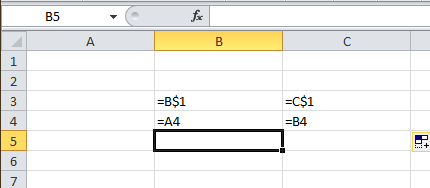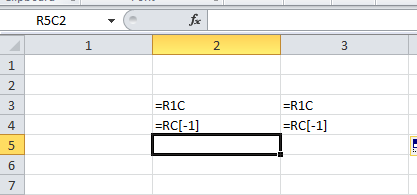The A1 style of referencing is is easy to understand as it refers to cells using letters for the column references numbers for row references. This is intuitive – maybe because that’s how we learnt about coordinates at school.

This isn’t the only way of referring to cells however. You may be familiar with named ranges, which allow us to assign a name to range (of one or more cells). We can then use that name in formulae to refer to the range. Referring to ranges using range names has some ‘interesting’ properties which can be both useful and unexpected if you aren’t familiar with them.

There is another way of referring to cells, which is the R1C1 style of formatting. This is an alternative to the A1 style and you can switch between them – Excel will display all the formulae in a workbook in whichever referencing style is currently active. Incidentally, range names work in R1C1 referencing just the same as with A1 referencing.

It may not be obvious why you would want to use R1C1 referencing – I for one find it less intuitive to use than A1 referencing, but it does have some interesting properties that we can exploit.

### What is R1C1 notation?

R1C1 effectively refers to cells by specifying the number of cells vertically and horizontally from the referencing cell. You can switch between A1 and R1C1 referencing in the Excel options (Excel Options>>Formulas under the “Working with formulas” heading). This can also be done using the keystroke combination Alt, F, T, [down arrow], Alt+R, Enter

Since this is a bit long winded, you could use a really simple macro to switch between the referencing styles easily – you could assign this to a button or a keystroke combination:

Sub ToggleR1C1()

If Application.ReferenceStyle = xlA1 Then

Application.ReferenceStyle = xlR1C1

Else

Application.ReferenceStyle = xlA1

End If

End Sub

In R1C1 notation, relative references (normally the default) have square brackets round the numbers. Negative numbers represent rows above and columns to the left of the referencing cell and positive numbers represent rows below and columns to the right of the referencing cell.

If there are no square brackets, the numbers represent the absolute row or column of the referenced cell in the worksheet. If there is no number, this means the referenced cell is on the same row or column (you can see an example in the screenshots below).

Consider a formula in B2 referring to A1 with the following combinations of absolute and relative referencing. On the left is the A1 reference and on the right is the R1C1 reference:

A1 reference R1C1 reference:

A1 =R[-1]C[-1]

\$A\$1 =R1C1

A\$1 =R1C[-1]

\$A1 =R[-1]C1

Here’s a simple example:

Here are two formulae in A1 style referencing copied across from column B to column C:Here are the same formulae in R1C1 Notation:As you can see, although the A1 style reference is different for the copied formula, the R1C1 style referencing is the same.

### So how is it useful?

The really useful thing about R1C1 notation is that a copied formula will be the same as the original with the exception of very rare circumstances.

This is different from A1 notation – if we have a formula in cell A2 that is “=A1” and copy this across to B2 the formula will be “=B1”.

The same formulae in R1C1 notation will be “=R[-1]C” in both cells -what I mean is that the cell reference appears exactly the same when copied across.

Since we and most practitioners consider it good practice to construct a formula that can be copied across a row, we need a way of checking this. Excel has some basic built in functionality to do this – Goto (hit F5) >> special >> row differences selects cells that contain formulae different from those in the ActiveCell.

As we know that copied formulae are the same in R1C1 notation, this can be used to check for copies. This is the basis for most spreadsheet mapping tools (these display the contents of a cell as either a label, constant, unique formula or copy of a unique formula from above, from the left or both).

### Now for the really cool part

Fairly frequently, I have a workbook where a large number of formulae are identical copies, but there are other formulae in between – usually subtotals. I may want to change all the identical formulae. Using R1C1 I can change them all in one go……

First switch to R1C1 notation
Copy one of the identical formulae (you should copy from the formula box using CTRL+C, don’t copy the cell itself)
Hit CTRL F (Find) and paste the formula into the Find dialog box

Now there are two options:

1) if you know what you want your revised formula to be in R1C1 notation, you can click the Replace tab and type it in the “Replace with” box and click the options, select the “Match entire cell contents” box and click the “Replace All” button.

2) If you don’t know the R1C1 formula and would rather work in A1 you can use the following to select all the identical formulae:

Click Find All
Select the first one in the list
Scroll down to the bottom and holding down the shift key, select the last one.
You now have all the identical formulae selected and you can switch back to A1 if you prefer to amend your formula. To enter the same formula in all the selected cells, hold down the CTRL key when you hit enter.

You’ll find that once you get to grips with it, R1C1 can enable you to do things you can’t do any other way – feel free to share your thoughts and experiences!

To easily switch between R1C1 and A1 style notation, you can use the shortcut included in our free add-in nXt – shortcut key combinations can be found after installation by clicking on the “Show shortcuts” button. Get the add-in by clicking below: